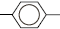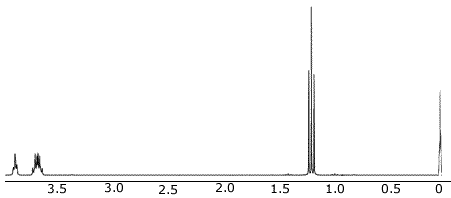1H Nuclear Magnetic Resonance Spectroscopy Tutorial

Key Concepts

• Nuclear Magnetic Resonance Spectroscopy, or NMR Spectroscopy, can be used to identify any isotope unless the isotope has both an even number of protons and an even number of neutrons.
• The nuclei of many elements, such as 1H, spin generating a magnetic field.
When placed in a strong external field they align themselves either with or against the field.
When the sample is irradiated with radio waves the energy absorbed corresponds to the difference between these two magnetic alignments.
• 1H NMR Spectroscopy (proton nuclear magnetic resonance spectroscopy) is used to identify the structure of organic (carbon) compounds.

1H NMR spectra provide information about:

1. The Number of Signals: each chemically different proton in a structure is also magnetically different.
CH3 groups are chemically different to CH2 groups and to CH groups.
eg, CH3CH2CH2Cl contains 3 chemically different groups of protons:
CH3
CH2 covalently bonded to Cl
2. The Relative Areas of Each Signal: the strength of the NMR signal is proportional to the number of protons giving rise to that signal, or, the area under the absorption curve is proportional to the number of protons.
3. The Position of the Signal with respect to an internal standard (chemical shift): tetramethylsilane, (CH3)4S or TMS, is often used as an internal standard since almost all proton signals appear downfield from the TMS signal.
On the δ scale, the TMS signal is 0 ppm, and on the τ scale, the TMS signal is 10 ppm. The two scales are related in that δ + τ = 10.
Tables of chemical shifts are derived from measurements of a large number of samples and represent a "normal" range.
Proton signals affected by highly electronegative elements are shifted further downfield.
4. The Influence of One Proton on Adjacent Protons (magnetic coupling or spin-spin splitting): neighbouring protons in chemically different groups may either reinforce or subtract from the effective force of the applied field, the net effect of this is to split the proton signal.
The proton signal will be split into n + 1 peaks by n adjacent protons.
Chemically Different Protons
Number of Peaks in a Signal
n + 1
Name Given
to Signal
0 0 + 1 = 1 singlet
1 1 + 1 = 2 doublet
2 2 + 1 = 3 triplet
3 3 + 1 = 4 quartet
4 4 + 1 = 5 quintet

No ads = no money for us = no free stuff for you!

Example: Number of Signals

How many signals are predicted to be in the 1H NMR spectrum of ethanol?
1. Draw the structural formula for ethanol, C2H5OH.
 H| H| H- C - C -O-H |H |H
2. Identify the groups of chemically different protons.
 H| H| H- C - C -O-H |H |H
There are 3 groups of protons:
• the protons in the CH3 group

• the protons in the CH2 group

• the proton in the O-H group
3. Predict the number of signals

Number of signals = number of chemically different groups of protons = 3 signals

Do you know this?

Play the game now!

Example: Signal Area

Predict the relative signal area in the 1H NMR spectrum of ethanol, CH3-CH2-OH:
signal area is proportional to the number of protons in each chemically different group.

Chemical Group Number of Protons
in Group
Number
of Groups
Total Number
of Protons
CH3 3 1 3
CH2 2 1 2
OH 1 1 1

The area under the CH3 signal will be 3 times greater than the area under the OH signal and 3/2 times greater than the area under the CH2 signal.

In order of increasing area under the signal:

OH < CH2 < CH3

Do you understand this?

Take the test now!

Example: Chemical Shift

Using the data provided below, predict the positions of each of the signals in ethanol, CH3-CH2-OH.

Proton Type δ (ppm) τ (ppm)
R-CH3 0.9 9.1
R-CH2-R 1.3 8.7
R3-CH 2.0 8.0
C=C-H 4.6 - 6.0 4.0 - 5.4
C≡C-H 2 - 3 7 - 8
C=C-CH2- 1.7 - 2.2 7.8 - 8.3
C-OH 1 - 6 4 - 9
R-CH2-OH 3.6 6.4
H-C=O 9 - 10 0 - 1
-COOH 10.5 - 12 -2 - -0.5
R-NH2 1- 5 4 - 9H 6 - 8.5 1.5 - 8.5

For ethanol, CH3CH2OH, we expect 3 proton signals corresponding to each of the 3 chemically different groups of protons.
Using the table of δ values above, we can get some idea of the likely chemical shifts for each proton type, keeping in mind that the chemical shifts of protons adjacent to the electronegative oxygen atom will be shifted downfield.

 H| H| H- C - C -O-H |H |H

 CH3 ~1 ppm CH2 ~3.6 ppm OH ~4 ppm

Can you apply this?

Join AUS-e-TUTE!

Take the exam now!

Example: Magnetic Coupling (Spin-Spin Splitting)

Predict the number of peaks associated with each signal in the 1H NMR spectrum of ethanol, CH3-CH2-OH.

CH3 group is adjacent to a CH2 group so the signal for CH3 will be split into 2 + 1 = 3 peaks (a triplet).

CH2 group is adjacent to a CH3 and an OH group, 4 protons in total, so the signal will be split into 4 + 1 = 5 peaks (a quintet).

OH group is adjacent to a CH2 group so will be split into 2 + 1 = 3 peaks (a triplet).

Can you apply this?

Join AUS-e-TUTE!

Take the exam now!

Example: 1H NMR Spectrum of Ethanol

Use the 1H NMR spectrum of ethanol shown below to determine the probable structure of the ethanol molecule.Number of signals: (0 ppm is TMS reference) 1.3 ppm triplet 3.6 ppm quintet 3.8 ppm triplet 3 signals indicates there are 3 chemically different groups of protons in the structure. Signal Area: 1.3 ppm area=3 3.6 ppm area=2 3.8 ppm area=1 Number of protons in each group in order of increasing chemical shift is proportional to 3 : 2 : 1 Identify proton types from signal area and chemical shift: 1.3 ppm CH3 3.6 ppm CH2 3.8 ppm OH Determine the position of each group in the structure from the number of peaks in each signal: CH3 triplet indicates that the CH3 is adjacent to 2 protons, ie, CH2 group. CH2 quintet indicates that this group is adjacent to 4 protons(one CH3 group and the OH group). OH triplet indicates it is adjacent to 2 protons, ie, CH2 group. Construct the probable condensed structural formula: CH3CH2OH

Can you apply this?

Join AUS-e-TUTE!

Take the exam now!